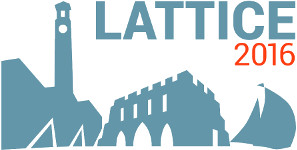#The 34th International Symposium on Lattice Field Theory (Lattice 2016)

Jul 24 – 30, 2016
Highfield Campus, University of Southampton
Europe/London timezone

## Numerical Analysis of Discretized ${\mathcal N}=(2,2)$ SYM on Polyhedra

Jul 26, 2016, 4:30 PM
20m
Building 67 Room 1027 (Highfield Campus, University of Southampton)

### Building 67 Room 1027

#### Highfield Campus, University of Southampton

Talk Physics Beyond the Standard Model

### Speaker

Dr Syo Kamata (Keio University)

### Description

We investigate the two-dimensional $\mathcal{N}=(2,2)$ supersymmetric Yang-Mills (SYM) theory on the discretized curved space both from theoretical and numerical points of view. We first show that the number of the supersymmetry of the continuum $\mathcal{N}=(2,2)$ SYM theory on any curved manifold is enhanced to two by considering an appropriate $U(1)$ gauge backgrounds associated with the $U_{V}(1)$ symmetry. We then show that the generalized Sugino model, which was proposed in our previous work, can be identified to the discretization of the SUSY enhanced theory, where one of the supersymmetries is kept and the other one is restored in the continuum limit. The $U_{A}(1)$ anomaly of the continuum theory is maintained also in the discretized theory as an unbalance of the number of the fermions. We study the discretized supersymmetric model based on the numerical Monte-Carlo simulation. In the process, we propose a novel phase-quenched approximation, which we call the anomaly-phase-quench approximation". We find that the Ward-Takahashi (WT) identity expected from the analytical study realizes in the model and there is no sign-problem by adopting the anomaly-phase-quench method. The result is the first numerical observation for the supersymmetric lattice model on the curved space with generic topologies.

### Primary author

Dr Syo Kamata (Keio University)

### Co-authors

Prof. Kazutoshi Ohta (Meiji Gakuin University) Prof. So Matsuura (Keio University) Prof. Tatsuhiro Misumi (Akita University, Keio University)

 Slides